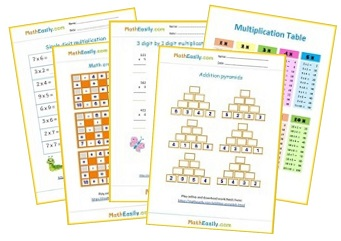﻿ 100+ Free Multiplication Games ONLINE Practice

Math Games > Math multiplication games

# 100+ Free Multiplication Games ONLINE Practice

Play free multiplication games online. These interactive times tables games help kids to master multiplication fast and easily. You can play these math multiplication online games for free.

All our interactive multiplication games for kids contain also worksheets with an answer key. You can use them in the classroom or at home. Enjoy multiplication practice online!

## Free multiplication games ONLINE

#### Multiplication dice games

Roll a pair of dice, multiply these 2 numbers, check your answer. Play fun multiplication games online.
Play now

### Single digit multiplication

Play single digit multiplication games online. More than 81 exercises available. Download fun multiplication worksheets.
Play now

### Multiplication pyramids

Play multiplication pyramid games. Download free multiplication worksheets. Online multiplication practice for kids.
Play now

### Multiplication flash cards online

Online multiplication flash cards 0-12 help kids to learn multiplication easily. More than 160 tasks available.
Play now

### Multiplication puzzles

Learn multiplication easily with this free online multiplication games. After completing all 6 multiplication tasks, a nice picture will be displayed.
Play now

### Multiplication arrays games

What problem does this multiplication array represent? Play multiplication games online.
Play now

### Printable multiplication games for kids

To help our kids to learn multiplication easily I designed this collection of free printable multiplication games in PDF.

## Free online multiplication games for kids

These free online multiplication games are full of nice pictures, kids will have fun while learning multiplication online.

### Multiplication crosswords

Play interactive multiplication games: solve multiplication crossword puzzles. Print out multiplication worksheets.

Play now

### Multiplication chart

Interactive multiplication chart games + printables.

Play now

### 2 digit by 1 digit multiplication

Learn how to multiply two by one digit numbers. Learning multiplication games for kids.

Play now

### Multiplication comparisons

Compare results of the multicplication. This math game improves multiplication and comparison skills.

Play now

### 3 digit by 1 digit multiplication

Multiply three digit by 1 digit numbers. Play multiplication games online, download worksheets.

Play now

Play now

### 3 digit by 2 digit multiplication

Practice three digit by two digit multiplication online. Online multiplication practice for 4th grade.

Play now

Play now

## Online math multiplication games for fun

Download free printable multiplication games and worksheets in PDF below. These worksheets help you to practice multiplication. Most of them contain also the answer key. You will find more worksheets under individual online multiplication games listed on this page or browse all our free multiplication worksheets here.## Interactive multiplication games FREE

Free online multiplication games for kids improve multiplication skills.

Because these interactive multiplication games and online math puzzles are full of nice pictures, students will have fun. Our free multiplication games stimulate students' interest and curiosity.

Kids can play our free multiplication games online. No hurry with answers, there is plenty of time in our fun multiplication games.

## Multiplication online games

These multiplication online games are self-checking multiplication activities that kids can use and play right on my website! There's nothing to download, and students can practice this on any device or any web browser.

I also created a few simple PDFs for each multiplication game with a link to the page that you can send to parents or print out for extra practice at home.Free Math Worksheets